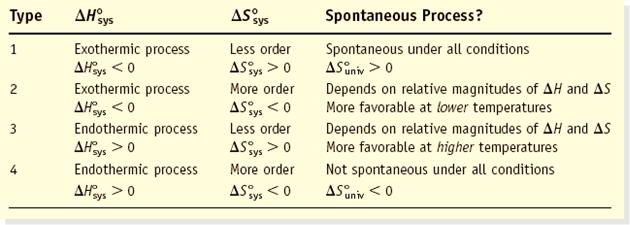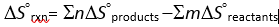# Entropy a state function

Chemical Thermodynamics: Entropy- a state function

Entropy is a thermodynamic property which is a measure of the disorder of a system. This means that more disorganized a system is, the higher its entropy.The entropy of a substance increases with temperature. Accordingly, an increase in molecular complexity results in an increase in S.Entropies of ionic solids depends on coulombic attractions. In most cases, entropy becomes greater in size when a true solid or liquid close down in a solvent.

In conclusion, S (gases) > S (liquids) > S (solids)

Remember that –∆H˚sys is proportional to ∆S˚surr and an exothermic process has ∆S˚surr> 0.

The second law of thermodynamics states that for an isolated or a closed adiabaticor System + Surroundings.

Suniv= ∆Ssys + ∆Ssurr> 0

When a system reaches equilibrium, both forwardand the reverse reaction are non- spontaneous; because there is no driving force. Therefore,

At equilibrium, ∆Suniv= ∆Ssys + ∆Ssurr= 0

Ssystem = 0 at 0 K

Table 1: Predicting spontaneity and non-spontaneity of a systemEntropy, unlike energy, is non-conservative because it is always increasing or remains constant but never decreases. This means that the universe is always becoming disorganized and is approaching a chaotic state. The generation of entropy is because of the presence of irreversibility. Thus, the more high the entropy generation the higher the irreparability and, accordingly, the lowest the efficiency of a device since a reversible system is the most efficient system.

Entropy is a state function which is independent of the path or history of the system. The Clausius inequality states that:

∆S ≥dq/T

In an isolated system,dQ = 0,which means that if the process occurs reversibly dS = 0 and irreversibly dS> 0.Figure : P-V graph of an expansion system

From the P-V graph above A: isothermal expansion, B:adiabatic expansion, C:isochoric processSince the temperature T is constant, the two quantities of entropy along A and B+C are equal showing that change in entropy is equal while heat is not.

Hence, Entropy change = Entropy Transfer + Entropy GenerationHere,m and n are the appropriate coefficients in the balanced chemical equation.

Consider 2 H2(g) + O2(g) —> 2 H2O(liq)

∆So = 2 So (H2O) – [2 So (H2) + So (O2)]

∆So = 2 mol (69.9 J/K•mol) – [2 mol (130.7 J/K•mol) + 1 mol (205.3 J/K•mol)]

∆So = -326.9 J/K

Therefore, 3 mol of gas give 2 mol of liquid which means that there is a decrease in entropy.

Alternatively, for a phase change where temperature of a system remains constant, the inequality changes to an absolute notation:

∆S = dq/T

For H2O (liq) —> H2O(g)

∆H = q = +40,700 J/mol